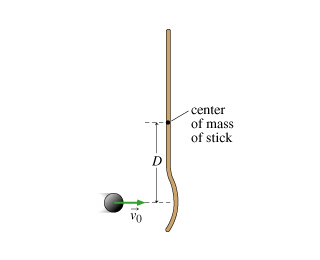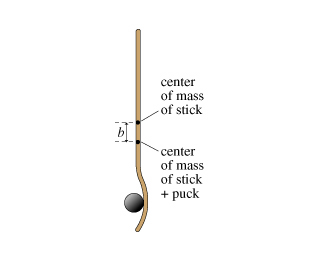# Problem: A hockey stick of mass ms and length L is at rest on the ice (which is assumed to be frictionless). A puck with mass mp hits the stick a distance D from the middle of the stick. Before the collision, the puck was moving with speed v0 in a direction perpendicular to the stick, as indicated in the figure. The collision is completely inelastic, and the puck remains attached to the stick after the collision.1. Find the speed vf of the center of mass of the stick+puckcombination after the collision.Express vf in terms of the followingquantities: v0, mp, ms, and L.2. After the collision, the stick and puck will rotate about their combined center of mass. How far is this center of mass from the point at which the puck struck? In the figure, this distance is (D - b). (Part B 1 figure)3. What is the angular momentum Lcm of the system before the collision, with respect to the center of mass of the final system?Express Lcm in terms of the given variables.4. What is the angular velocity ω of the stick+puck combination after the collision? Assume that the stick is uniform and has a moment of inertia I0 about its center.Your answer for ω should not contain the variable b.5. Which of the following statements are TRUE?1) Kinetic energy is conserved.2) Linear momentum is conserved.3) Angular momentum of the stick+puck is conserved about the center of mass of the combined system.4) Angular momentum of the stick+puck is conserved about the(stationary) point where the collision occurs.

###### FREE Expert Solution

1.

Linear momentum:

$\overline{){\mathbf{p}}{\mathbf{=}}{\mathbf{m}}{\mathbf{v}}}$

According to the conservation of linear momentum:

pi = pf

msv0 + mp(0) = (ms + mp)vf

97% (323 ratings)###### Problem Details

A hockey stick of mass ms and length L is at rest on the ice (which is assumed to be frictionless). A puck with mass mp hits the stick a distance D from the middle of the stick. Before the collision, the puck was moving with speed v0 in a direction perpendicular to the stick, as indicated in the figure. The collision is completely inelastic, and the puck remains attached to the stick after the collision.1. Find the speed vf of the center of mass of the stick+puckcombination after the collision.

Express vf in terms of the followingquantities: v0, mp, ms, and L.

2. After the collision, the stick and puck will rotate about their combined center of mass. How far is this center of mass from the point at which the puck struck? In the figure, this distance is (D - b). (Part B 1 figure)

3. What is the angular momentum Lcm of the system before the collision, with respect to the center of mass of the final system?

Express Lcm in terms of the given variables.

4. What is the angular velocity ω of the stick+puck combination after the collision? Assume that the stick is uniform and has a moment of inertia I0 about its center.

5. Which of the following statements are TRUE?

1) Kinetic energy is conserved.
2) Linear momentum is conserved.
3) Angular momentum of the stick+puck is conserved about the center of mass of the combined system.
4) Angular momentum of the stick+puck is conserved about the(stationary) point where the collision occurs.